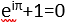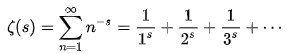The Riemann Hypothesis, Part 1 – the Zeta Function

Okay. This is the most arcane topic yet. There is no easy way into the Riemann Hypothesis, or RH, as it is affectionately called. I’m testing the limits of my understanding even more than I have before.

If you want to pass on this one, please do. However, if you know enough math to get the beauty of Euler’s equation, you can follow what I’m going to say. Euler’s equation is:If you find yourself unable to look at that equation without a rapidly growing sense of awe, stick with me. What I say may ultimately be nonsense, but you will follow my reasoning (or lack of it!). BTW- the Euler equation is just a test. It has nothing to do with what I’m going to say.

Here we go.

In a paper in 1859, Bernhard Riemann discussed a function of an infinite series called the Zeta function. Here it is:The squiggle at the front is the symbol for Zeta. The equation is read as “Zeta of s equals the summation, for all natural numbers (n) from 1 to infinity, of 1 over ‘n to the s power’”. The beginning of this infinite sum is shown in the last part. Of course, being infinite, it continues forever. There are ways to determine the answer to certain kinds of infinite sums, and the Zeta function is one of those, so finding the answer for any particular value of s is not a problem. (I know it is for you and me, but mathematicians can do it.)

The interesting part is finding patterns in those answers as you solve for more and more values of s, and particularly patterns in the values of s for which the answer is zero. The values of s for which the Zeta function equals zero are called solutions of the function. You’ll remember this from algebra.

Example (x^2 means 'x squared'): take the function f(x)= x^2-5x+6, then only look for those answers for which f(x) is equal to 0.  x^2-5x+6=0 is true only if x=2 or x=3, so f(x)= x^2-5x+6 has two solutions, 2 and 3. Why does the answer zero matter more than all the other possible answers to f(x)= x^2-5x+6? It depends on the function and what you are using it for. For the Zeta function, the zeros, and the s values that produce them, matter a lot in number theory.

The significance of the Zeta function here, is that it’s an analytic function that is connected to how the prime numbers are distributed in the list of natural numbers. (Who saw THAT coming...I didn’t!) This connects analysis, which includes continuous functions, with number theory in a very deep way. Rigorously connecting the continuous to the discrete in math (sort of like analog to digital) is important, as it gives the tools of each some power to understand the other.

The prime numbers are to all appearances randomly distributed as you count up through the natural numbers. Riemann discovered (among other things) boundaries to the number of primes occurring (and their distribution) within the list of consecutive natural numbers up to any chosen number.

He used solutions to the Zeta function to do this.

Remember, solutions to the Zeta function are those values of s for which the function Zeta of s is equal to zero. Now, this is not one of your grandmother’s polite finite polynomial functions, this is an infinite analytic function, and there is an infinite number of solutions. Yikes!

However, the solutions can be grouped in to two categories, called trivial and non-trivial. The trivial solutions are all the negative even numbers: s = -2, s = -4, s = -6, etc., which all give zero as the value for Zeta of s.

The non-trivial solutions are, well, not trivial. First of all, while n is always an integer, s can be any damn number you can think of, AND --- it doesn’t have to be a real number. Most numbers aren’t real. Many, many, many more numbers are complex. They have a real part and an imaginary part and have the general form a + bi, where a and b are real numbers and i is the square root of minus 1. The real numbers are just the subset of complex numbers for which b is zero and so the imaginary half (bi) is zero. a+0=a, a real number.

Riemann noticed that every non-trivial solution he found was of the form ½ + bi. In every solution the real number part was equal to ½ no matter what the imaginary part was. For reasons he didn’t make clear (though there are several good ones), he conjectured that all non-trivial solutions to the Zeta function have real part ½. This is the Riemann Hypothesis.

But he couldn’t prove it. Neither has anyone else in the more than a century and a half since then.

Who cares? Well, it turns out that if the RH is true, a whole lot of other math theorems are true also. There seem to be countless math papers that start with something like “Assume the Riemann Hypothesis is true…” and go on for thousands of words, or what passes for words to a mathematician. If the RH is NOT true, all of this comes crashing down.

I have some ideas about this. I’ll go into those further along. (In case you're wondering, no, I'm not going to try to prove it!)

Hugh Moffatt
Portland, Oregon
March 12, 2020